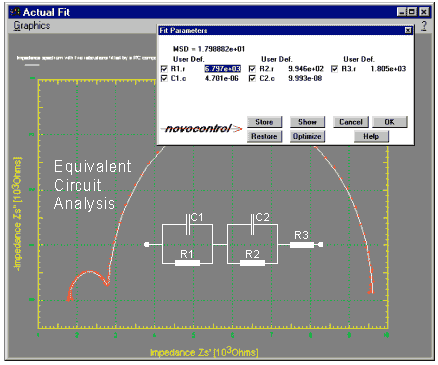# Equivalent Circuit AnalysisWinFIT equivalent circuit analysis supports definition of arbitrary RLC networks in combination with any user defined complex function such as sin(w*(var1+j*var2)).

Equivalent circuit analysis is used to describe the impedance measured on a material or system under test by an equivalent circuit made up of electronic components. The components are often resistors, capacitors and inductors.

For a given circuit, the task is to find the optimal value for each circuit component, so that the equivalent circuit impedance function matches the measured impedance is good as possible.

With the WinFIT equivalent circuit analysis function, arbitrary models can be defined and the best set of values (parameters) is automatically detected. In addition, arbitrary components described by any complex impedance function can be defined.

An example for corrosion analysis is shown below. The measurement shows the impedance of paint layer which is separate by an oxide layer due to corrosion  from a metal carrier plate. Each layer can be modeled in first order approximation by a parallel RC circuit as shown below. From the fitted data, non destructive information on both layers like e.g. the layer thickness can be determined.